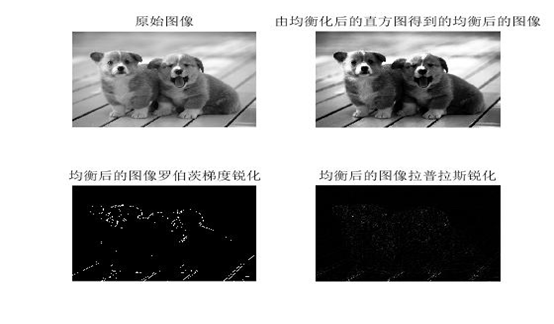# 图像增强 数字图像处理

## 数字图像处理1--图像增强技术

2015-10-31 15:32:34 samkieth 阅读数 15909
• ###### matlab图像复原和图像增强技术

系统的学习和掌握matlab图像复原和图像增强技术

18课时 182分钟 550人学习 刘昱显
免费试看

# 空间域图像增强

g(x, y) =T[f (x, y)]定义了空间域。

f(x,y)是原图像，g(x,y)是处理后的图像

T是作用于f的操作，定义在(x,y)的邻域

r是f(x,y)在任意点(x,y)的灰度级，s是g(x,y)在任意点(x,y)的灰度级

## 1.点运算

### 1.1灰度变换

#### 1.1.1图像反转

[0,L-1]为图像的灰度级。作用：倒转图像的灰度，适用于增强嵌入于图像暗色区域的白色或灰色细节，特别是当黑色面积占主导地位时。

c是常数,r ≥ 0。

#### 1.1.3.幂次变换

c和γ正常数

γ <1 提高灰度级，在正比函数上方，使图像变亮

γ >1 降低灰度级，在正比函数下方，使图像变暗

c=γ=1时简化为正比变换。#### 1.1.5.灰度切割#### 1.1.6.位图切割

这种方法通过对特定位提高亮度，改善图像质量。

较高位（如前4位）包含大多数视觉重要数据。

较低位（如后4位）对图像中的微小细节有作用。

分解为位平面，可以分析每一位在图像中的相对重要性。### 1.2直方图处理

#### 1.2.1.直方图两种定义：

nk是图像中灰度级为rk的像素个数

rk 是第k个灰度级，k = 0,1,2,…,L-1

p(k)= nk

n 是图像的像素总数

nk是图像中灰度级为rk的像素个数

rk是第k个灰度级，k = 0,1,2,…,L-1

#### 1.2.3.直方图均衡化（待补充）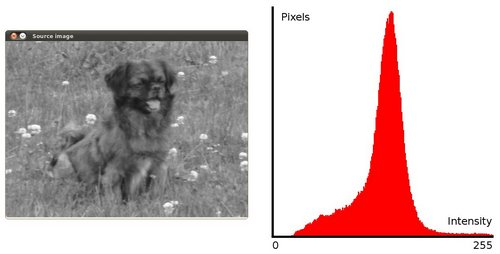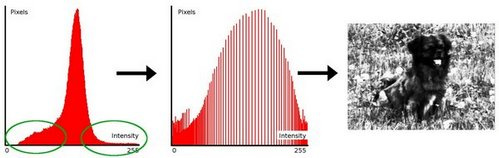均衡化指的是把一个分布 (给定的直方图) 映射 到另一个分布 (一个更宽更统一的强度值分布), 所以强度值分布会在整个范围内展开.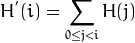•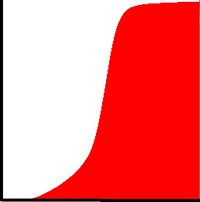• 最后, 我们使用一个简单的映射过程来获得均衡化后像素的强度值:
•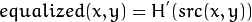## 2.邻域去噪### 2.1.平滑滤波器

#### 2.1.1线性滤波器:均值滤波器#### 2.1.2非线性滤波器:统计排序滤波器(中值滤波器最有代表性)

统计排序滤波器基于滤波器所在图像区域中像素的排序，由排序结果决定的值代替中心像素的值

中值滤波器： 用像素领域内的中间值代替该像素

最大值滤波器：用像素领域内的最大值代替该像素

最小值滤波器：用像素领域内的最小值代替该像素

主要用途：去除噪声

计算公式：R = mid {zk | k = 1,2,…,n}

最大值滤波器

主要用途：寻找最亮点

计算公式：R = max {zk | k = 1,2,…,n}

最小值滤波器

主要用途：寻找最暗点

计算公式：R = min {zk | k = 1,2,…,n}

中值滤波的原理

像素由小到大排列，用模板区域内像素的中间值，作为结果值。

R = mid {zk | k = 1,2,…,n}

强迫突出的亮点（暗点）更象它周围的值，以消除孤立的亮点（暗点）### 2.2.锐化滤波器二阶微分滤波器－拉普拉斯算子

一阶微分滤波器－梯度算子

#### 2.2.1拉普拉斯算子(1)用于拉普拉斯模板中心系数为负

(1)用于拉普拉斯模板中心系数为正#### 2.2.2梯度算子微分过滤器的原理

∇f ≈ |z6 – z5| + |z8 – z5|

∇f ≈ [(z9 – z5)2 + (z8 – z6)2]1/2

≈ |z9 – z5| + |z8 – z6|

Roberts交叉梯度算子

Prewitt梯度算子

Sobel梯度算子# 频域图像增强

## 数字图像和数字图像处理

2016-08-15 21:29:57 xlx921027 阅读数 5075
• ###### matlab图像复原和图像增强技术

系统的学习和掌握matlab图像复原和图像增强技术

18课时 182分钟 550人学习 刘昱显
免费试看

# Digital Image Processing[数字图像和数字图像处理]

## 1.  景象：人眼所看到的外部世界。

人类看世界的生理功能：由于光线照射到物体上，经过不发光的物体反射形成的反射光线或发光物体发出的光线射入人们的眼睛中。这些光信号在视网膜上转成神经电信号，然后神经电信号通过神经纤维传送到大脑皮层中，使人们看到外部世界

## 2.  图象：记录下来的景象，是对视觉信息的记录和展现 。

要形成一幅图像,必须有两个因素:一个是景象,另一个是电磁波。以光速传播，所以事件的成像是在瞬间进行的。电磁波与物体的相互作用决定了图像中呈现出的物体的几何形状。忧郁电磁波的波长不同，电磁波与物质的相互作用形式也不同。{γ射线区, x射线，UV， 可见光， IR， 微波， FM波 }

#### 4.  数字图像：将连续图像进行数字化后，用一个矩阵表示的图像## 6.  数字图像处理系统： 能够完成图像处理和分析任务的系统。主要有：图像输入设备，执行分析与处理图像的计算机及图像处理机，输出设备及存储系统中的图像数据库等。

7图像处理硬件系统主要由图像采集系统、图像处理系统和图像输出系统三部分组成。

(1)数字图像采集系统原始的图像数据是通过图像采集部件进入计算机的,即图像采集部件是采集原始的模拟图像数据,并将其转换成数字信号,计算机接受到图像的数字信号后,将其存入内存储区。

(2) 数字图像处理系统在数字图像处理系统中,图像处理工作是由计算机完成的,计算机的扩展槽上插有带帧存储器的采集卡,图像处理的过程通常包含从帧存储器提取数据到计算机内存、处理内存中的图像数据和送数据回图像帧存储器三个步骤。对于直接使用内存的采集卡,则只需和内存进行数据交换,计算机的内存越大,CPU的运算速度越快,图像处理的速度也就越快。

(3)数字图像输出系统图像的输出是图像处理的最终目的。图像输出有两种基本形式:一种是根据图像处理的结果作出判断,例如质量检测中的合格与不合格,输出不一定以图像作为最终形式,而只需作出提示供人或机器进行选择。这种提示可以是计算机屏幕信息,或是电平信号的高低,样的输出往往用于成熟研究的应用上。另一种则是以图像为输出形式,它包括中间过程的监视以及结果图像的输出。图像输出方式有屏幕输出、打印输出和视频硬拷贝输出。

## 数字图像处理实验三图像增强

2018-07-23 21:08:29 weixin_39569242 阅读数 4035
• ###### matlab图像复原和图像增强技术

系统的学习和掌握matlab图像复原和图像增强技术

18课时 182分钟 550人学习 刘昱显
免费试看

（1）了解图像增强的目的及意义，加深对图像增强的

感性认识，巩固所学的图像增强的理论知识和相

关算法。

（2）熟练掌握直方图均衡化和直方图规定化的计算过

程。

（3）熟练掌握空域滤波中常用的平滑和锐化滤波器。

（4）熟练掌握低通和高通滤波器的使用方法，明确不

同性质的滤波器对图像的影响和作用。

（5）掌握最简单的伪彩色变换方法。

（1）任意选择几幅图像，对其进行平滑处理，用

不同的平滑模板，对结果进行分析。

（2）任意选择几幅图像，对其进行中值滤波，用

不同的滤波模板对结果进行分析。

（3）任意选择几幅图像，对其进行梯度锐化，选

择不同的阈值参数，观察图像有何变化。

（4）对图像进行伪彩色变换，比较彩色增强后的

图像与原图像有何不同。

(1)平滑滤波----邻域平均法

• 代码：

I=rgb2gray(I);

J=imnoise(I,'salt & pepper',0.03);       %加均值为0，方差为0.03的椒盐噪声G=imnoise(I,'gaussian',0.03);             %加均值为0，方差为0.03的高斯噪声。

A=fspecial('average',[4,4]); %4x4均值滤波

A1=fspecial('average',[6,6]); %6x6均值滤波

A2=fspecial('average',[8,8]); %8x8均值滤波

A3=fspecial('average',[10,10]); %10x10均值滤波

J1= imfilter(J,A);  %imfilter均值滤波函数

J2= imfilter(J,A1);

J3= imfilter(J,A2);

J4= imfilter(J,A3);

G1= imfilter(G,A);

G2= imfilter(G,A1);

G3= imfilter(G,A2);

G4= imfilter(G,A3);

figure(1)

subplot(2,3,1),imshow(I),title('原图像');

subplot(2,3,4),imshow(I),title('原图像');

subplot(2,3,2),imshow(J),title('加入椒盐噪声');         %显示有椒盐噪声图像

subplot(2,3,5),imshow(G),title('加入高斯噪声');         %显示有高斯噪声图像

subplot(2,3,3),imshow(J1),title('4×4均值滤波')；%显示有椒盐噪声图像的滤波

subplot(2,3,6),imshow(G1),title('4×4均值滤波'); %显示有高斯噪声图像的滤波

figure(2);

subplot(2,3,1),imshow(I),title('原图像');

subplot(2,3,2),imshow(J),title('加入椒盐噪声');  %显示有椒盐噪声图像

subplot(2,3,4),imshow(J1),title('4×4');

subplot(2,3,3),imshow(J2),title('6×6');

subplot(2,3,5),imshow(J3),title('8×8');

subplot(2,3,6),imshow(J4),title('10×10');

figure(3)

subplot(2,3,1),imshow(I),title('原图像');

subplot(2,3,2),imshow(G),title('加入高斯噪声');       %显示有高斯噪声图像

subplot(2,3,4),imshow(G1),title('4×4');

subplot(2,3,3),imshow(G2),title('6×6');

subplot(2,3,5),imshow(G3),title('8×8');

subplot(2,3,6),imshow(G4),title('10×10');

• 结果：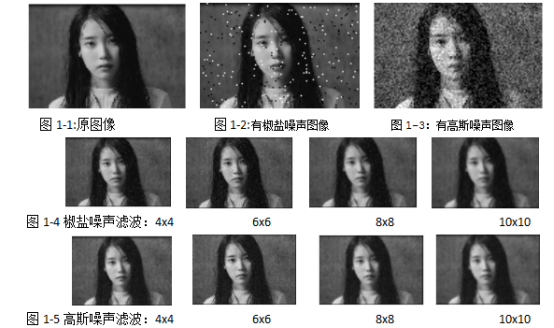• 分析：
1. 平滑滤波的作用是对高频分量进行削弱或消除，增强图像的低频分量
2. 领域平均法是线性运算、中值滤波是非线性运算
3. 在该题中加的椒盐噪声、高斯噪声方差均为0.03，可以由图1-2、图1-3看出原图像在加入两种噪声之后，画质都有所损失。但是椒盐噪声只是在原图像的某些地方存在，而高斯噪声几乎是分布在原图像的每个地方，造成了图像比之前模糊
4. 椒盐噪声：又称脉冲噪声，它随机改变一些像素值，是由图像传感器，传输信道，解码处理等产生的黑白相间的亮暗点噪声。椒盐噪声往往由图像切割引起；
5. 高斯分布：也称正态分布，有均值和方差两个参数，均值反应了对称轴的方位，方差表示了正态分布曲线的胖瘦。高斯分布是最普通的噪声分布
6. 平滑滤波的模板需要选择合适，模板选择太小，噪声无法滤除，模板选择太大，造成了过度处理，图像会变得逐渐模糊，不管是椒盐噪声、还是高斯噪声如图1-4、1-5模板逐渐增大，图像逐渐模糊。

（2）平滑滤波----中值法

• 代码：

I=rgb2gray(I);

temp = I;

I = double(I);

%Robert梯度

w1 = [-1 0; 0 1];

w2 = [0 -1;1 0];

G1 = imfilter(I,w1,'corr','replicate');

G2 = imfilter(I,w2,'corr','replicate');

G = abs(G1)+abs(G2);

figure(1);

subplot(2,2,1);imshow(temp),title('原图像');

subplot(2,2,2);imshow(abs(G1),[]),title('w1滤波');

subplot(2,2,3);imshow(abs(G2),[]),title('w2滤波');

subplot(2,2,4);imshow(G,[]),title('Robert梯度');

%sobel梯度

w11 = fspecial('sobel');

w21 = w11';

G11 = imfilter(I,w11);

G21 = imfilter(I,w21);

G1 = abs(G11)+abs(G21);

figure(2);

subplot(2,2,1);imshow(temp),title('原图像');

subplot(2,2,2);imshow(G11,[]),title('水平sobel');

subplot(2,2,3);imshow(G21,[]),title('竖直sobel');

subplot(2,2,4);imshow(G1,[]),title('sobel');

%拉普拉斯滤波

w12 = [0 -1 0;-1 4 -1;0 -1 0];

L12 = imfilter(I,w12,'corr','replicate');

figure(3);

subplot(1,2,1);imshow(temp),title('原图像');

subplot(1,2,2);imshow(abs(L12),[]);

• 结果：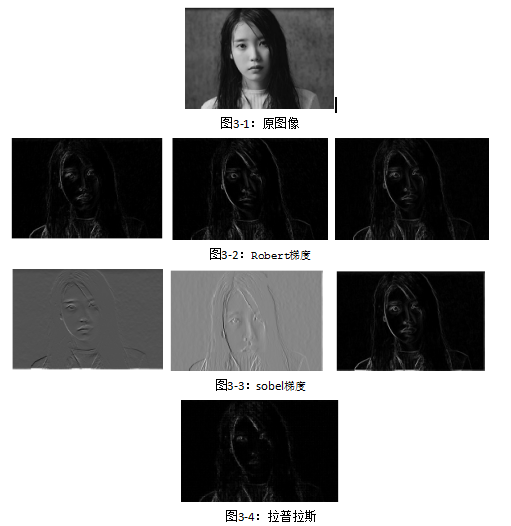• 分析：
1. 锐化滤波器可以消除或减弱图像的低频分量从而增强图像中物体的边缘轮廓信息，使得除边缘以外的像素点的灰度值趋向于零
2. 我们要将图像类型从uint8转换为double.因为锐化模板计算时常常使输出产生负值，如果采用无符号的 uint8 型， 则负位会被截断
3. 图3-2Robert梯度分别以w1和w2为模板，w1对接近正45 度边缘有较强响应： w2对接近负45 度边缘有较强响应,对原图像进行滤波就可得到GI和G2,最终的Robert交叉梯度图像（b）为：G = ｜G1｜ + ｜G2｜.
4. 图3-3，第一个计算了一幅图像的竖直梯度，第2个计算了一幅图像的水平梯度 它们的和可以作为完整的Sobel梯度
5. 拉普拉斯滤波输出图像中的双边缘，拉普拉斯锐化对一些离散点有较强的响应
6.
7. （3）伪彩色变换
• 代码：

gray=rgb2gray(im);

I=double(gray);

[m,n]=size(I);

L=256;

for i=1:m

for j=1:n

if I(i,j)<=L/2    %绿色通道

R(i,j)=0;

G(i,j)=2*I(i,j);

B(i,j)=L;

else if I(i,j)<=L/4   %蓝色通道

R(i,j)=0;

G(i,j)=L;

B(i,j)=-4*I(i,j)+2*L;

else if I(i,j)<=3*L/2   %红色通道

R(i,j)=2*I(i,j)-2*L;

G(i,j)=L;

B(i,j)=0;

else

R(i,j)=L;

G(i,j)=-4*I(i,j)+4*L;

B(i,j)=0;

end

end

end

end

end

for i=1:m

for j=1:n

rgbim(i,j,1)=R(i,j);

rgbim(i,j,2)=G(i,j);

rgbim(i,j,3)=B(i,j);

end

end

rgbim=rgbim/256;

figure(1);

subplot(1,2,1),imshow(gray),title('原图');

subplot(1,2,2),imshow(rgbim),title('伪彩色变换');

• 结果：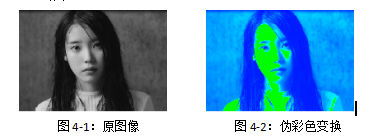• 分析：
1. 伪彩色处理是指通过将每一个灰度级匹配到彩色空间上的一点，将单色图像映射为一副彩色图像的彩色图像
2. 最小的灰度值0映射为红色，中间的灰度值L/2映射为绿色，最高的灰度值L映射为蓝色

## 数字图像处理图像增强技术

2016-10-21 16:57:03 fish_mai 阅读数 9753
• ###### matlab图像复原和图像增强技术

系统的学习和掌握matlab图像复原和图像增强技术

18课时 182分钟 550人学习 刘昱显
免费试看

4.1 概述和分类g(x, y) = EH[f(x, y)]

（1）g(x, y)值仅取决于f(x, y)值，以s和t代表f和g在(x, y)位置处的灰度值，则：t = EH(s) EH就是灰度变换。
（2）g(x, y)值不仅取决于f(x, y)值，而且取决于邻域内的象素值。称为模板操作

（1）将图象从图象空间转换到频域空间所需的变换
（2）增强操作如下4.2 空域变换增强

（1）直接用EH操作变换
（2）借助f的直方图进行变换
（3）借助对一系列图像间的操作进行变换
4.2.1 直接灰度变换
1. 灰度求反

2. 增强对比度3. 动态图象压缩

4. 灰度切分4.2.2 直方图处理1. 直方图均衡化

(1) EH(s)在0 <= S <= L-1范围内是1个单值单增函数；
(2)s和t的值域相同（1）对原始图像进行灰度均衡化（2）规定需要的直方图，并计算能使规定的直方（3）将第一个步骤得到的变换翻转过来，即将原始直方图对应映射到规定的直方图4.2.3 图象间运算

g(x, y) = f(x, y) - h(x, y)4.3 空间滤波增强
4.3.1 原理与分类（1）将模板在图中漫游，并将模板中心与图中某个象素位置重合；
（2）将模板上系数与系数与末班下对应象素相乘
（3）将所乘的结果相加
（4）将和（模板的输出响应）赋值给原始图4.3.2 平滑滤波器
1. 线性平滑滤波器2. 非线性平滑滤波器（G2）

（1）将模板在图中漫游
（2）读取灰度值
（3）将灰度值从小到大排成1类；
（4）找出这些值里排在中间的1个；
（5）这个中间值赋值给模板中心。

（1）最大值滤波器：百分比去最大，可检测图像中最亮的点。
（2）最小值滤波器：百分比最小，检测图像中最暗的点。
（3）中值滤波器：百分比取50%。4.3.2 锐化滤波器1. 线性锐化滤波器（G3）——最常用的线性高通滤波器Highpass = Original - Lowpass

High boost = A*Original - Lowpass = (A-1) * Original + Highpass
（1）当A = 1，就是普通的高通滤波器。
（2）当A > 1，原始图的一部分与高通图相加，恢复了部分高通滤波时丢失的低频分量
2. 非线性锐化滤波器（G4）4.4 频域增强
4.4.1 原理和分类（1）计算待增强图的傅里叶变换，得到F(u, v);
（2）将其余1个（根据需要设计的）转移函数H(u, v)相乘；
（3）再将结果傅里叶反变换可以得到增强的图

4.4.2 理想低通滤波器"振铃（ring）"现象2. 巴特沃斯低通滤波器（Butterworth）4.4.3 高通滤波器
1. 理想高通滤波器2. 巴特沃斯高通滤波器（Butterworth）4.4.4 带通和带阻滤波器4.4.5 同态滤波器4.5 局部增强

（1）可借助将图象分成子图像再对每个子图像增强。

（2）也可在对整幅图增强时直接利用局部信息以达到不同局部不同增强的目的4.6 彩色增强
4.6.1 伪彩色增强（从无彩色到有彩色）

1. 亮度切割2. 从灰度到彩色的变换3. 频域滤波4.6.2 真彩色增强（从有彩色到有彩色）

（1）在HSI模型中，亮度分量与色度分量是分开的。
H：色调（Hue）和S：饱和度——构成色度分量；I：密度——构成亮度分量

（2）在HSI模型中，色调和饱和度的概念与人类感知系统联系。

（1）RGB分量转换为HSI分量
（2）利用灰度图增强的方法增强其中的I分量
（3）再将结果转换为RGB分量表示

## 数字图像处理--图像增强MATLAB程序

2018-08-20 08:42:43 iefenghao 阅读数 9834
• ###### matlab图像复原和图像增强技术

系统的学习和掌握matlab图像复原和图像增强技术

18课时 182分钟 550人学习 刘昱显
免费试看

### 1 图像增强--灰度

//灰度
I=rgb2gray(P);
subplot(231);imshow(I,[]);title('原始图像');
subplot(234);imhist(I);title('由原始图像得到的直方图');
subplot(232);imshow(J);title('由新直方图得到的新图像');
K=histeq(I);
subplot(233);imshow(K);title('由均衡化后的直方图得到的均衡后的图像');
subplot(236);imhist(K);title('均衡化后的直方图');


### 实验结果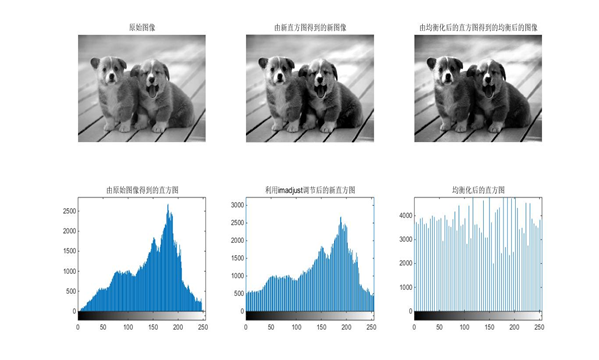### 2 图像增强--平滑

//平滑
I=rgb2gray(P);
subplot(221);imshow(I);title('原始图像');
I1=imnoise(I,'salt & pepper');
subplot(222);imshow(I1);title('加入椒盐噪声后的图像');
h1=ones(3,3)/9;
h2=ones(5,5)/25;
K1=imfilter(I1,h1);
K2=imfilter(I1,h2);
%subplot(334),imshow(I),title('原始图像');
%subplot(337),imshow(I),title('原始图像');
subplot(223),imshow(K1,[]),title('加入椒盐噪声后3*3平均滤波');
subplot(224),imshow(K2,[]),title('加入椒盐噪声后5*5平均滤波');

I2=imnoise(I,'gaussian',0,0.005);
figure;
subplot(221);imshow(I);title('原始图像');
subplot(222);imshow(I2);title('加入高斯噪声后的图像');
h1=ones(3,3)/9;
h2=ones(5,5)/25;
K1=imfilter(I2,h1);
K2=imfilter(I2,h2);
subplot(223),imshow(K1,[]),title('加入高斯噪声后3*3平均滤波');
subplot(224),imshow(K2,[]),title('加入高斯噪声后5*5平均滤波');

figure;
subplot(321);imshow(I1);title('加入椒盐噪声后的图像');
subplot(322);imshow(I2);title('加入高斯噪声后的图像');
J1=medfilt2(I1);
J3=medfilt2(I2);
J2=medfilt2(I1,[5 5]);
J4=medfilt2(I2,[5 5]);
subplot(323),imshow(J1),title('加入椒盐噪声后3*3窗口中值滤波');
subplot(324),imshow(J3),title('加入椒盐噪声后5*5窗口中值滤波');
subplot(325),imshow(J2),title('加入高斯噪声后3*3窗口中值滤波');
subplot(326),imshow(J4),title('加入高斯噪声后5*5窗口中值平均滤波');


### 实验结果

加入高斯噪声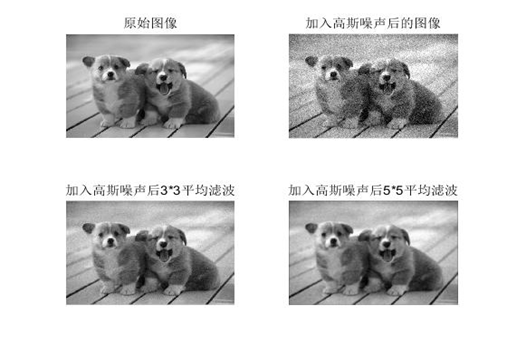加入椒盐噪声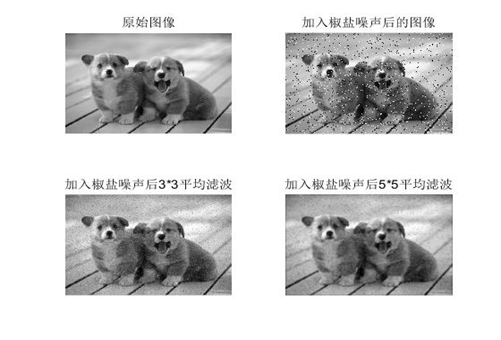平滑中值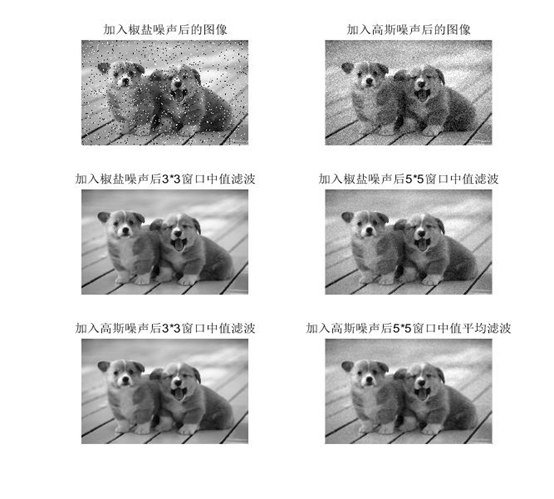### 3 图像处理--锐化

//锐化
I=rgb2gray(P);
subplot(221);imshow(I,[]);title('原始图像');
K=histeq(I);
subplot(222);imshow(K);title('由均衡化后的直方图得到的均衡后的图像');
BW=edge(K,'roberts',0.1);
subplot(223);imshow(BW);title('均衡后的图像罗伯茨梯度锐化');
h=[0 -1 0;-1 4 -1;0 -1 0];
J=imfilter(I,h);
subplot(224);imshow(J);title('均衡后的图像拉普拉斯锐化');


### 实验结果